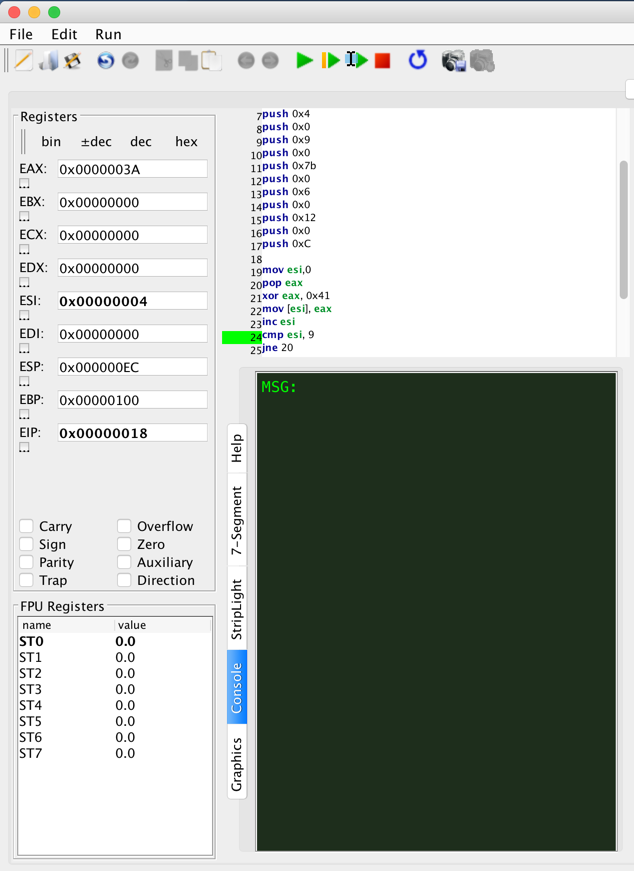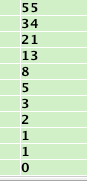Project 1x: Assembly Code Challenges (30 points)

Challenge 1: Secret Message (10 pts)

The code loads encrypted values onto the stack, decrypts them to place a secret message in ASCII into memory, and then erases the memory.

In the bottom-center pane of Jasmin, click Console to see the message, as shown below.Use Jasmin to run the code until it has decrypted the message, but has not erased it yet, and capture a whole-desktop image of the decrypted RAM contents. Save the image as "Proj 1xa from YOUR NAME".

Type the message in the body of your email message.

Challenge 2: Fibonacci Numbers (20 pts.)

Write an assembly language program to display the first 10 Fibonacci numbers, as shown below:Each Fibbonacci number is the sum of the first two previous ones, and the first two are:

F0 = 0
F1 = 1

F2 = F1 + F0 = 1
F3 = F2 + F1 = 1 + 1 = 2
F4 = F3 + F2 = 2 + 1 = 3
...

Tip: You can learn how to use loops here:

Capture a whole-desktop image showing your results, and attach an ASM file of your code, saved from Jasmin.

Save the image as "Proj 1xb from YOUR NAME".